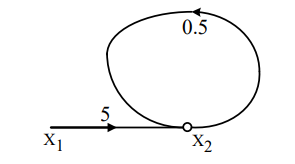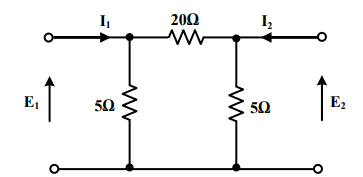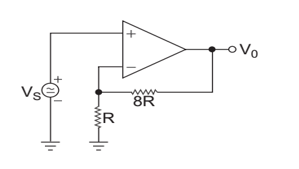Engineering Jobs   »   ELECTRICAL GATE QUIZ

# GATE’22 EE: Daily Practices Quiz 09-Aug-2021

Each question carries 2 marks
Negative marking: 0.66 mark

Total Questions: 05
Total marks: 10
Time: 12 min.

Q1. The transfer function of a linear system is the
(a) ratio of the output, V_0 (t) and input V_i (t).
(b) ratio of the derivatives of the output and the input.
(c) ratio of the Laplace transforms of the output and that of the input with all initial conditions zero.
(d) none of these

Q2. In the signal flow graph shown in figure X_2 = TX_1 where T, is equal to(a) 2.5
(b)5
(c) 5.5
(d)10

Q3. The admittance parameter Y_12 in the 2-port network in figure is(a) -0.2 mho
(b) 0.1 mho
(c) -0.05 mho
(d) 0.05 mho

Q4. A dc shunt generator when driven without connecting field winding shows as open circuit terminal voltage of 12 V. When field winding is connected and excited the terminal voltage drops to zero because
(a) field resistance is higher than critical resistance
(b) there is no residual magnetism in the field circuit
(c) field winding has got wrongly connected
(d) there is a fault in armature circuit

Q5. In the op amp circuit, V0 is given by(a) 8Vs
(b) -8Vs
(c) 2Vs
(d) 9Vs

SOLUTIONS

S1. Ans.(c)
Sol. The transfer function of a linear system is the ratio of Laplace transform of the output and input with all initial conditions zero.

S2. Ans.(d)
Sol. X_2 = TX_1
From signal flow graph: X_2/X_1 =5/Δ=5/(1-0.5)=10.

S3. Ans.(c)
Sol. Y_12=-1/20=-0.05 ℧

S4. Ans.(c)
Sol. The wrongly connected field circuit can destroy the residual magnetism.

S5. Ans.(d)
Sol. V_o=V_s (1+8R/R)=9V_s

Sharing is caring!